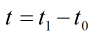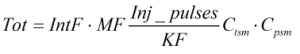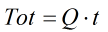# C052 – Liquid Totalisation – Pulse Output Meter

## Description

This calculates the totals for pulse output based meters where the metered fluid is a liquid.

IP Petroleum Measurement Manual Part Ⅻ – Static and Dynamic Measurement of Light Hydrocarbon Liquids – Section 1: Calculation Procedures

Kelton calculation reference C052

KIMS calculation reference K134

Kelton Calculation C203 – Totalisation Conversion

Kelton Calculation C264 – Flow Rate – Pulse Output Meter

## Options

### Method

• Injected frequency
• Injected pulses

This option is used to select whether the total will be calculated from the flow rate if frequency is chosen or calculated directly using the number of pulses measured.

### Enter elapsed time as

• Duration
• Start and end time

This option is used to select whether the time over which the totals are to be calculated is entered as a duration or as a start and end time.

### Totals

• Use start and end totals

This option allows the user to enter displayed totals for the start and end of the totalisation process for comparison with the calculated results.

The remaining options are the same as are found in K132 – Liquid Flow Rate – Pulse Output Meter

## Calculation

### Duration

If the duration is calculated this is done by:Where t1 = End time t0 = Start time

### Totals

If injected pulses is selected the totals are calculated from the equation:If injected frequency is selected the totals are calculated from flow rate by:Where IntF = Interpolation factor MF = Meter Factor Inj_pulses = Injected pulses KF = K-factor Ctsm = Correction for temperature on steel of meter Cpsm = Correction for pressure on steel of meter Q = Flow rate t = Duration

Back